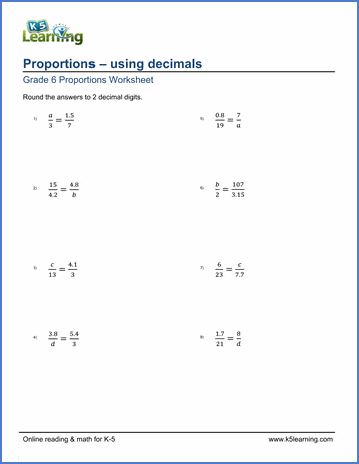# Proportions Worksheets

## Proportions and ratios

The use of proportions is a practical math skill that students often struggle with.  Our proportions worksheets review whole number and decimal proportions as well as provide simple proportion word problems.

## Proportions

Simple proportions 1 / 3 = x / 9
Proportions (answers rounded to 1 decimal) 38 / 2 = 14 / x
Proportions (decimals) 17.3 / x = 11 / 15.7

Proportion word problems Word problems
Proportion word problems (decimals) Word problems
Ratio word problems Word problems